Types of Triangles Scroll to the bottom for the triangle area calculator

All triangles have 3 sides and 3 angles which always add up to 180°.

The Triangle Inequality Theorem states that:
The longest side of any triangle must be
less than the sum of the other 2 sides.

Triangles are classified in 2 ways-

1) By the number of equal sides they have:
• scalene - all 3 sides have different lengths
• isosceles - 2 sides have equal lengths
• equilateral - all 3 sides are equal

2) By the types of angles they have:
• acute triangle - all 3 angles are acute (less than 90°)
• right triangle - has one right angle (a right angle = 90°)
• obtuse triangle - has one obtuse angle (an obtuse angle is greater than 90° and less than 180°).

When these 2 categories are combined, there are 7 possible triangles:
• acute scalene (diagram A)
• right scalene (B) - all right triangles are scalene (except diagram E).
• obtuse scalene (C)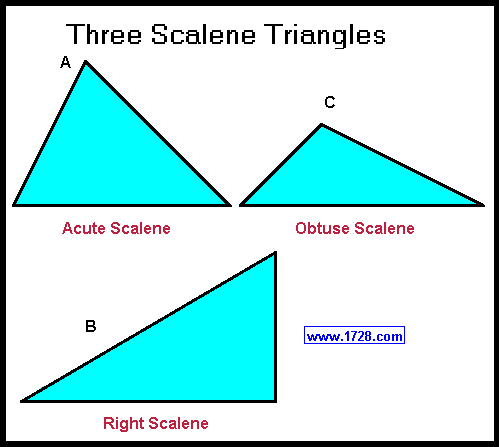• acute isosceles (diagram D)
• right isosceles (E) also known as a 45° 45° 90° triangle.
• obtuse isosceles (F)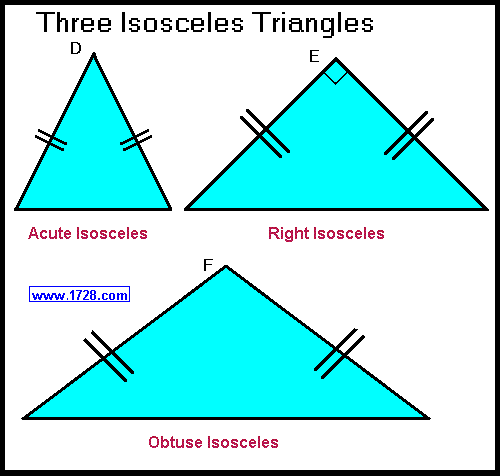• equilateral (G) all sides are equal and each angle = 60°, making this the only equiangular triangle.
Since all 3 angles are less than 90° all equilateral triangles are acute triangles.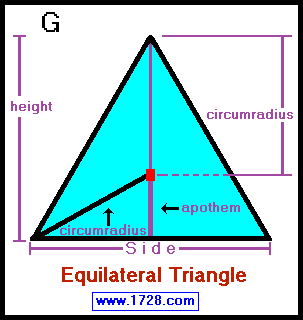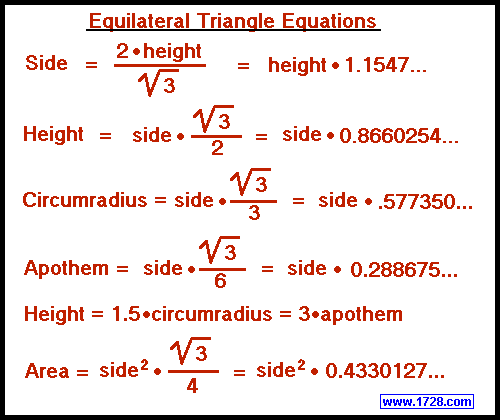There is one more type of triangle that is worth mentioning.
An oblique triangle is any triangle that is not a right triangle.

3 Triangle Area Formulas

1) The most well-known triangle area formula is multiplying the length of the base by the height (also called the altitude), and dividing that by 2.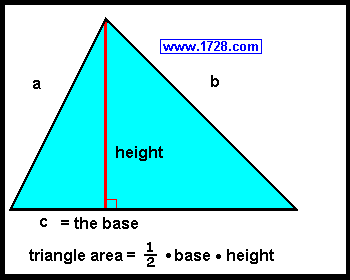2) If you know the length of all 3 sides of a triangle, you can calculate the area by using Heron's Formula (sometimes called Hero's Formula).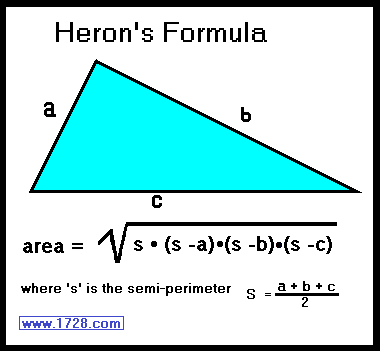First we have to define a triangle's perimeter which is (side a + side b + side c).
A triangle's semi-perimeter (or 's') is one half of the perimeter or to put it another way:

semi-perimeter = (side a + side b + side c) ÷ 2

Example: A triangle has side a = 4, side b = 5 and side c = 6. What is its area?
The perimeter = 4 + 5 + 6 = 15.
The semi-perimeter is one half of this or 7.5
Using Heron's formula,

area = square root (s • (s - a) • (s - b) • (s - c))

area = square root (s • (s - 4) • (s - 5) • (s - 6))
area = square root (7.5 • (7.5 - 4) • (7.5 - 5) • (7.5 - 6))
area = square root (7.5 • (3.5) • (2.5) • (1.5))
area = square root (98.4375)
area = 9.921567416...

 * * * * * * * * * * * * * * * * * * * * * * * * * * * * * By algebraic manipulation we can state Heron's Formula in two more ways.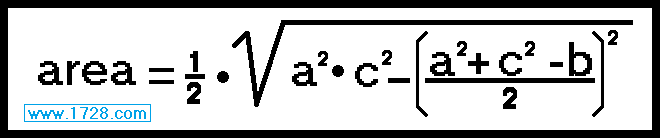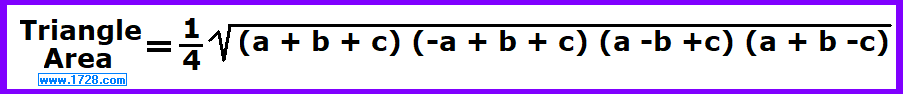* * * * * * * * * * * * * * * * * * * * * * * * * * * * *

3) If you know 2 sides and an included angle, there is a third formula for determining triangle area.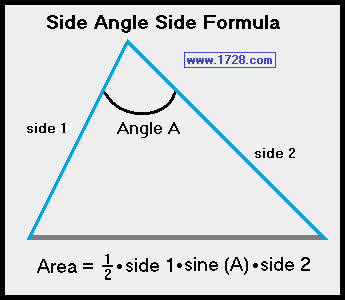This formula applies to all triangles and not just right triangles.
Area = ½ • side 1 • sine (A) • side 2

This is an easy formula to prove. First, we use the traditional formula:
Area = ½ • height • base     then we can substitute side 2 for the base:
Area = ½ • height • side 2

Since sine (A) = height / side 1     then height = side 1 • sine(A)
and then area = ½ • side 1 • sine (A) • side 2

Triangle Area Calculator

This calculator determines triangle area by using any of the 3 methods above.

Significant Figures >>>